# Tomography Inversion using Tikhonov and Sparse Regularization¶

Important: Please read the installation page for details about how to install the toolboxes. $\newcommand{\dotp}{\langle #1, #2 \rangle}$ $\newcommand{\enscond}{\lbrace #1, #2 \rbrace}$ $\newcommand{\pd}{ \frac{ \partial #1}{\partial #2} }$ $\newcommand{\umin}{\underset{#1}{\min}\;}$ $\newcommand{\umax}{\underset{#1}{\max}\;}$ $\newcommand{\umin}{\underset{#1}{\min}\;}$ $\newcommand{\uargmin}{\underset{#1}{argmin}\;}$ $\newcommand{\norm}{\|#1\|}$ $\newcommand{\abs}{\left|#1\right|}$ $\newcommand{\choice}{ \left\{ \begin{array}{l} #1 \end{array} \right. }$ $\newcommand{\pa}{\left(#1\right)}$ $\newcommand{\diag}{{diag}\left( #1 \right)}$ $\newcommand{\qandq}{\quad\text{and}\quad}$ $\newcommand{\qwhereq}{\quad\text{where}\quad}$ $\newcommand{\qifq}{ \quad \text{if} \quad }$ $\newcommand{\qarrq}{ \quad \Longrightarrow \quad }$ $\newcommand{\ZZ}{\mathbb{Z}}$ $\newcommand{\CC}{\mathbb{C}}$ $\newcommand{\RR}{\mathbb{R}}$ $\newcommand{\EE}{\mathbb{E}}$ $\newcommand{\Zz}{\mathcal{Z}}$ $\newcommand{\Ww}{\mathcal{W}}$ $\newcommand{\Vv}{\mathcal{V}}$ $\newcommand{\Nn}{\mathcal{N}}$ $\newcommand{\NN}{\mathcal{N}}$ $\newcommand{\Hh}{\mathcal{H}}$ $\newcommand{\Bb}{\mathcal{B}}$ $\newcommand{\Ee}{\mathcal{E}}$ $\newcommand{\Cc}{\mathcal{C}}$ $\newcommand{\Gg}{\mathcal{G}}$ $\newcommand{\Ss}{\mathcal{S}}$ $\newcommand{\Pp}{\mathcal{P}}$ $\newcommand{\Ff}{\mathcal{F}}$ $\newcommand{\Xx}{\mathcal{X}}$ $\newcommand{\Mm}{\mathcal{M}}$ $\newcommand{\Ii}{\mathcal{I}}$ $\newcommand{\Dd}{\mathcal{D}}$ $\newcommand{\Ll}{\mathcal{L}}$ $\newcommand{\Tt}{\mathcal{T}}$ $\newcommand{\si}{\sigma}$ $\newcommand{\al}{\alpha}$ $\newcommand{\la}{\lambda}$ $\newcommand{\ga}{\gamma}$ $\newcommand{\Ga}{\Gamma}$ $\newcommand{\La}{\Lambda}$ $\newcommand{\si}{\sigma}$ $\newcommand{\Si}{\Sigma}$ $\newcommand{\be}{\beta}$ $\newcommand{\de}{\delta}$ $\newcommand{\De}{\Delta}$ $\newcommand{\phi}{\varphi}$ $\newcommand{\th}{\theta}$ $\newcommand{\om}{\omega}$ $\newcommand{\Om}{\Omega}$

This numerical tour explores the reconstruction from tomographic measurement with Sobolev and sparse regularization.

In :
addpath('toolbox_signal')


## Tomography and Regularization¶

We consider here tomography measurements $y = \Phi f_0 + w$, where $f_0$ is the (unknown) image to recover, and $w$ is an additive noise.

The tomography operator $\Phi$ is ill-posed, so it cannot be inverted.

We consider variational reconstruction methods, that finds a solution through a convex optimization: $$f^\star \in \text{argmin}_f \frac{1}{2}\|y-\Phi f\|^2 + \lambda J(f)$$

Where $J(f)$ is a prior energy. In this tour we consider a a Sobolev prior (the image is uniformly smooth) and a sparse wavelet prior (the image is expected to be compressible in a wavelet basis).

Note that the parameter $\lambda$ should be carefully chosen to fit the noise level.

## Tomography Acquisition over the Fourier Domain¶

The tomography operator computes the projection of the signal along rays. It corresponds to the Radon transform $$(T_\theta f)( t ) = \int_{\Delta_{\theta,t}} f(x) d x,$$ where $\Delta_{\theta,t}$ is the line $$\Delta_{\theta,t} = { x = (x_1,x_2) \::\: x_1 \cos(\theta) • x_2 \sin(\theta) = t }.$$

A tomography measurment computes $$\{ T_{\theta_i} f \}_{i=0}^{q-1}$$ for a small sub-set of $q$ orientation $\theta_i \in [0,\pi)$.

Set the number $q$ of rays used for the experiments.

In :
q = 32;


Size of the image, the number of pixels is $N=n^2$.

In :
n = 256;


The Fourier Slice Theorem shows that obtaining the measurements $T_{\theta} f$ is equivalent to computing slice of the 2D Fourier transform of $f$ along a ray of direction $\theta$ $$\hat T_{\theta} f(\omega) = \hat f( r \cos(\theta), r \cos(\theta) ).$$

In this tour, we thus consider a discrete Fourier version of the Tomography operator. It corresponds to sampling the Fourier transform of the image along discretized rays $\Omega$.

Compute the set of rays $\Omega$ by computing the mask $\xi$ so that $\xi(\omega)=1$ if $\omega \in \Omega$ and $\xi(\omega) = 0$ otherwise.

In :
Theta = linspace(0,pi,q+1); Theta(end) = [];
xi = zeros(n,n);
for theta = Theta
t = linspace(-1,1,3*n)*n;
x = round(t.*cos(theta)) + n/2+1; y = round(t.*sin(theta)) + n/2+1;
I = find(x>0 & x<=n & y>0 & y<=n); x = x(I); y = y(I);
xi(x+(y-1)*n) = 1;
end


In Matlab, the 0 frequency is in the upper-left corner.

In :
xi = fftshift(xi);


In :
clf;
imageplot(fftshift(xi));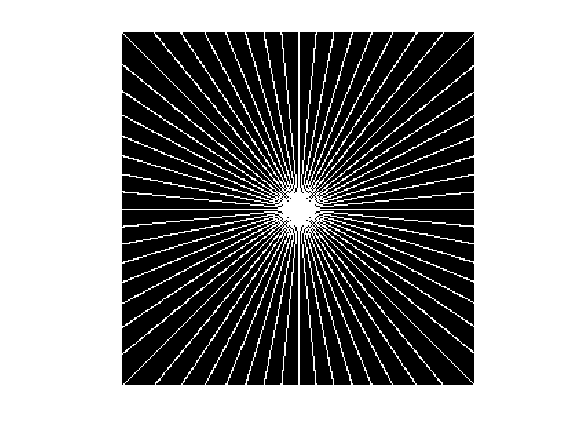Sampling the Fourier transform for points is equivalent to masking the Fourier transform $\hat f$ of $f$. We thus define our Fourier tomography measurements operator as $$\Phi f(\omega) = \hat f(\omega) \xi(\omega)$$ where $\hat f$ is the 2-D discrete Fourier transform of $f$.

Define a shortcut for the operator. Note the normalization of the FFT by $1/n$ to make it orthonormal.

In :
Phi  = @(f)fft2(f).*xi / n;


Load the clean high resolution image.

In :
name = 'mri';
f0 = rescale(f0);


We consider here noisy tomographic measurements. $$y = \Phi f_0 + w$$ where $w$ is a Gaussian white noise of variance $\sigma^2$.

Variance of the noise.

In :
sigma = .2;


Measurements.

In :
y = Phi(f0) + sigma*randn(n,n);


The pseudo inverse $\Phi^+$ is equal to the transposed operator $\Phi^*$. It corresponds to inverting the Fourier transform after multiplication by the mask. $$\Phi^* y = \Phi^+ y = \mathcal{F}^{-1}( y \xi ),$$ where $\mathcal{F}^{-1}(\hat f) = f$ is the inverse Fourier transform.

Define a shortcut for the transpose operator.

In :
PhiS = @(y)real(ifft2(y.*xi))*n;


Exercise 1

Compute and display the pseudo inverse reconstruction $\Phi^+ y$. What do you observe ?

In :
exo1()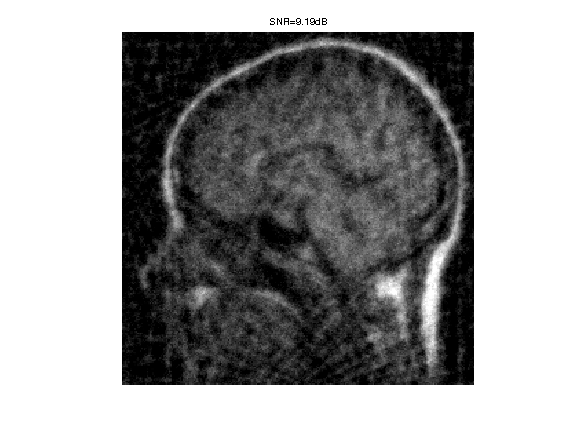In :
%% Insert your code here.


## Sobolev Regularization¶

To remove some noise while inverting the operator, we can penalize high frequencies using Sobolev regularization.

The Sobolev prior reads: $$J(f) = \frac{1}{2} \sum_x \|\nabla f(x)\|^2 = \frac{1}{2}\sum_{\omega} S(\omega) \|\hat f(\omega)\|^2$$ where $S(\omega)=\|\omega\|^2$.

Compute the Sobolev prior penalty $S$ (rescale to $[0,1]$).

In :
x = [0:n/2-1, -n/2:-1];
[Y,X] = meshgrid(x,x);
S = (X.^2 + Y.^2)*(2/n)^2;


We need to compute the solution of: $$f^\star \in \text{argmin}_f \frac{1}{2}\|y-\Phi f\|^2 + \lambda J(f)$$

Regularization parameter $\lambda$:

In :
lambda = 2;


Since both the prior $J$ and the operator $\Phi$ can be written over the Fourier domain, one can compute the solution to the inversion with Sobolev prior simply with the Fourier coefficients: $$\hat f^\star(\omega) = \frac{\hat y(\omega) \xi(\omega)}{ \xi(\omega) + \lambda S(\omega) }$$

Perform the inversion.

In :
fSob = real( ifft2( y .* xi ./ ( xi + lambda*S) ) )*n;


Display the result.

In :
clf;
imageplot(clamp(fSob), ['Sobolev inversion, SNR=' num2str(snr(f0,fSob),3) 'dB'] );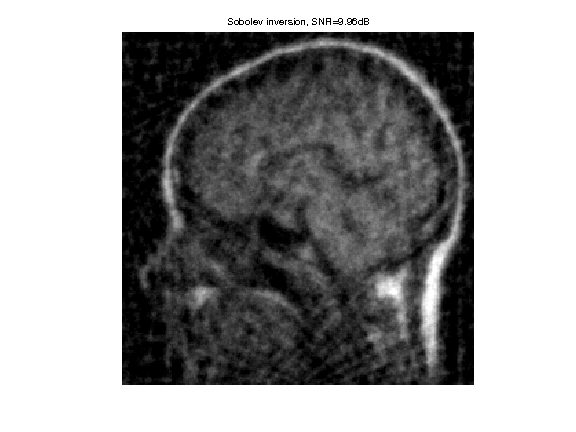Exercise 2

Find the optimal solution |fSob| by testing several value of $\lambda$.

In :
exo2()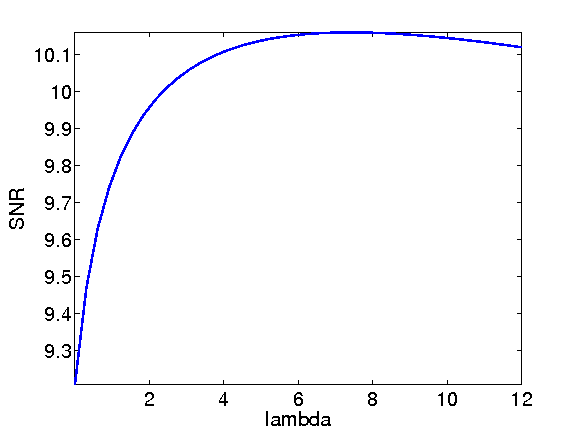In :
%% Insert your code here.


Display optimal result.

In :
clf;
imageplot(clamp(fSob), ['Sobolev inversion, SNR=' num2str(snr(f0,fSob),3) 'dB']);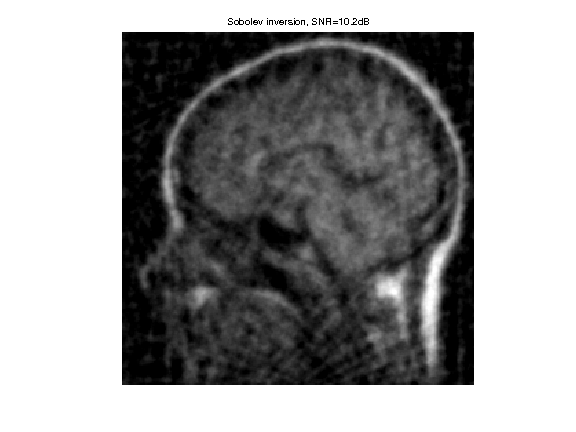## Soft Thresholding in a Basis¶

The soft thresholding operator is at the heart of $\ell^1$ minimization schemes. It can be applied to coefficients $a$, or to an image $f$ in an ortho-basis.

The soft thresholding is a 1-D functional that shrinks the value of coefficients. $$s_T(u)=\max(0,1-T/|u|)u$$

Define a shortcut for this soft thresholding 1-D functional.

In :
SoftThresh = @(x,T)x.*max( 0, 1-T./max(abs(x),1e-10) );


Display a curve of the 1D soft thresholding.

In :
clf;
T = linspace(-1,1,1000);
plot( T, SoftThresh(T,.5) );
axis('equal');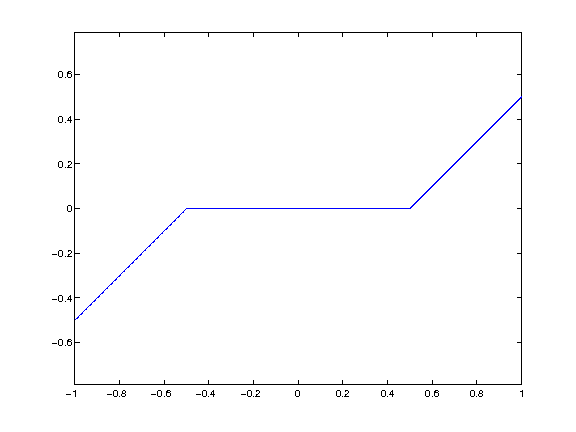Note that the function |SoftThresh| can also be applied to vector (because of Matlab/Scilab vectorialized computation), which defines an operator on coefficients: $$S_T(a) = ( s_T(a_m) )_m.$$

In the next section, we use an orthogonal wavelet basis $\Psi$.

We set the parameters of the wavelet transform.

In :
Jmax = log2(n)-1;
Jmin = Jmax-3;


Shortcut for $\Psi$ and $\Psi^*$ in the orthogonal case.

In :
options.ti = 0; % use orthogonality.
Psi = @(a)perform_wavelet_transf(a, Jmin, -1,options);
PsiS = @(f)perform_wavelet_transf(f, Jmin, +1,options);


The soft thresholding opterator in the basis $\Psi$ is defined as $$S_T^\Psi(f) = \sum_m s_T( \langle f,\psi_m \rangle ) \psi_m$$

It thus corresponds to applying the transform $\Psi^*$, thresholding the coefficients using $S_T$ and then undoing the transform using $\Psi$. $$S_T^\Psi(f) = \Psi \circ S_T \circ \Psi^*$$

In :
SoftThreshPsi = @(f,T)Psi(SoftThresh(PsiS(f),T));


This soft thresholding corresponds to a denoising operator.

In :
clf;
imageplot( clamp(SoftThreshPsi(f0,.1)) );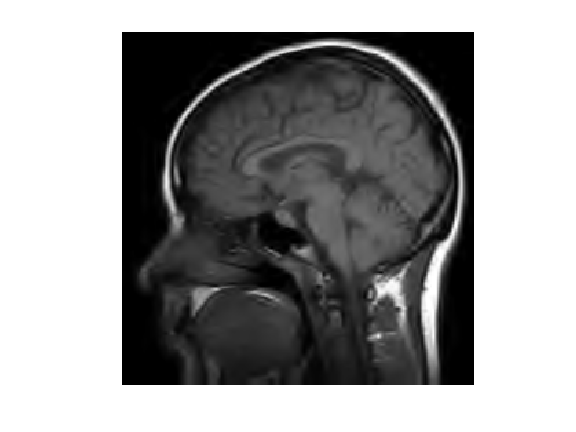## Sparse Regularization¶

To better reconstruct edges, we consider a sparsity regularization in an orthogonal basis $\{ \psi_m \}_m$: $$J(f)=\sum_m \vert \langle f,\psi_m \rangle\vert = \| \Psi^* f \|_1.$$

The inverse problem resolution thus require to solve: $$f^{\star} \in \text{argmin}_f \: E(f) = \frac{1}{2}\|y-\Phi f\|^2 + \lambda \sum_m \vert \langle f,\psi_m \rangle\vert.$$

Set up the value of the regularization parameter $\lambda$.

In :
lambda = 0.1;


To solve this non-smooth optimization problem, one can use forward-backward splitting, also known as iterative soft thresholding.

It computes a series of images $f^{(\ell)}$ defined as $$f^{(\ell+1)} = S_{\tau\lambda}^{\Psi}( f^{(\ell)} - \tau \Phi^{*} (\Phi f^{(\ell)} - y) )$$

For $f^{(\ell)}$ to converge to a solution of the problem, the gradient step size should be chosen as $$\tau < \frac{2}{\|\Phi^* \Phi\|}$$

Since $\Phi$ is an operator of norm 1, this must be smaller than 2.

In :
tau = 1.5;


Initialize the solution.

In :
fSpars = PhiS(y);


First step: perform one step of gradient descent of the energy $\|y-\Phi f\|^2$.

In :
fSpars = fSpars + tau * PhiS( y-Phi(fSpars) );


Second step: denoise the solution by thresholding.

In :
fSpars = SoftThreshPsi( fSpars, lambda*tau );


Exercise 3

Perform the iterative soft thresholding. Monitor the decay of the energy $E$ you are minimizing.

In :
exo3()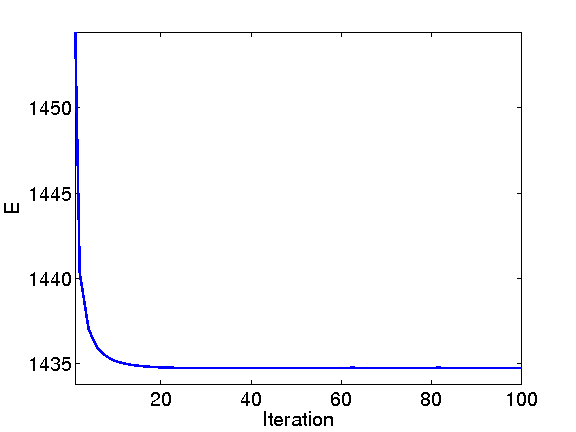In :
%% Insert your code here.


Display the result.

In :
clf;
imageplot(clamp(fSpars), ['Sparsity inversion, SNR=' num2str(snr(f0,fSpars),3) 'dB']);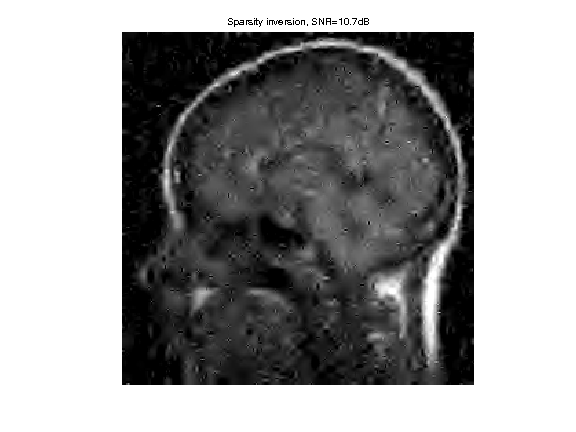Exercise 4

Try to find the best threshold $\lambda$. To this end, perform a lot of iterations, and progressively decay the threshold $\lambda$ during the iterations. Record the best result in |fBestOrtho|. armup terations

In :
exo4()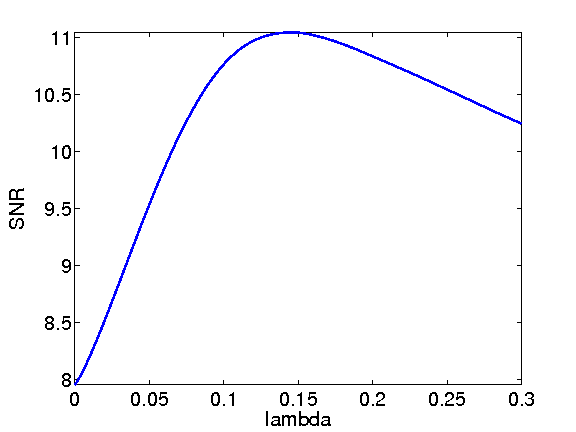In :
%% Insert your code here.


Display the result.

In :
clf;
imageplot(clamp(fBestOrtho), ['Sparsity in Orthogonal Wavelets, SNR=' num2str(snr(f0,fBestOrtho),3) 'dB']);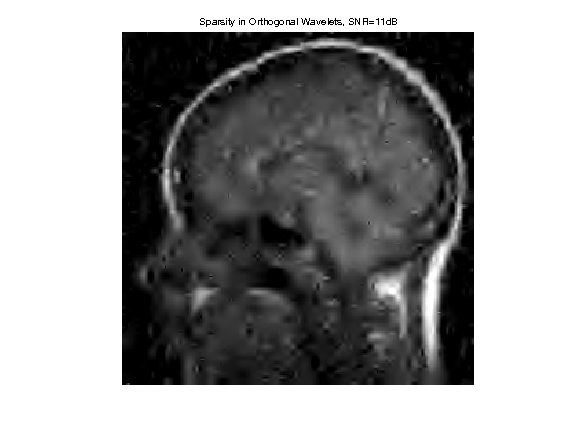## Translation Invariant Regularization¶

Denoising artefact are reduced by using a translation invariant wavelet frame.

Define new shortcut for the translation invariant wavelet transforms.

In :
options.ti = 1;
Psi = @(a)perform_wavelet_transf(a, Jmin, -1,options);
PsiS = @(f)perform_wavelet_transf(f, Jmin, +1,options);


Re-define the thresholding.

In :
SoftThreshPsi = @(f,T)Psi(SoftThresh(PsiS(f),T));


Exercise 5

Use the iterative thresholding but this time with the translation invariant wavelet transform. Find the best value of $\lambda$ and record the best result in |fBestTI|. armup terations

In :
exo5()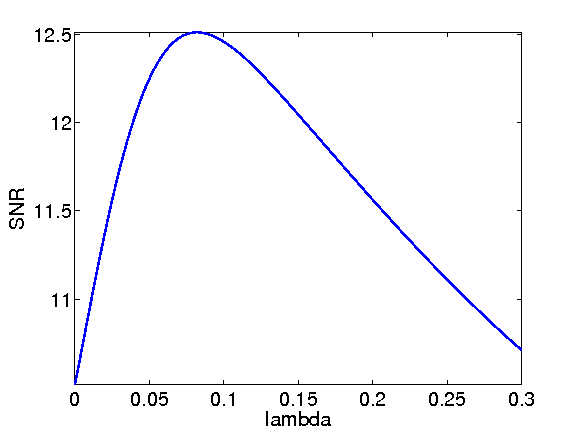In :
%% Insert your code here.


Display the result.

In :
clf;
imageplot(clamp(fBestTI), ['Sparsity in TI Wavelets, SNR=' num2str(snr(f0,fBestTI),3) 'dB']);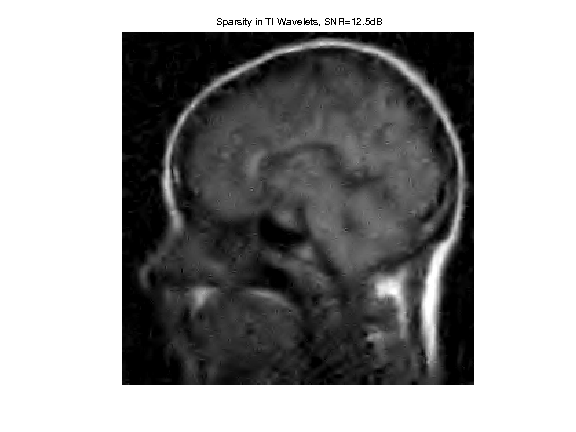## Medical Resonnance Imaging ¶

MRI acquisition directly gather Fourier measurement along a curve in the Fourier plane.

Create a spiral for the sampling. The parameter $\alpha$ controls the density near the origin.

In :
alpha = 3;
q = 30;
t = linspace(0,1.5,n*q*10);
x = round(.5*n*t.^alpha.*cos(2*pi*q*t)) + n/2+1;
y = round(.5*n*t.^alpha.*sin(2*pi*q*t)) + n/2+1;
I = find(x>0 & x<=n & y>0 & y<=n); x = x(I); y = y(I);
xi = zeros(n,n); xi(x+(y-1)*n) = 1;


Display the sampling pattern.

In :
clf;
imageplot(xi);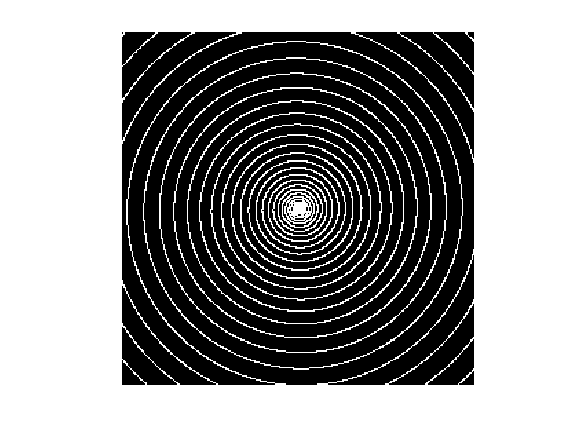Exercise 6

Compare Sobolev and Sparse reconstruction for MRI imaging. For a given number of Fourier sample, compare the quality of the reconstruction for different $\alpha$.

In :
exo6()

In :
%% Insert your code here.